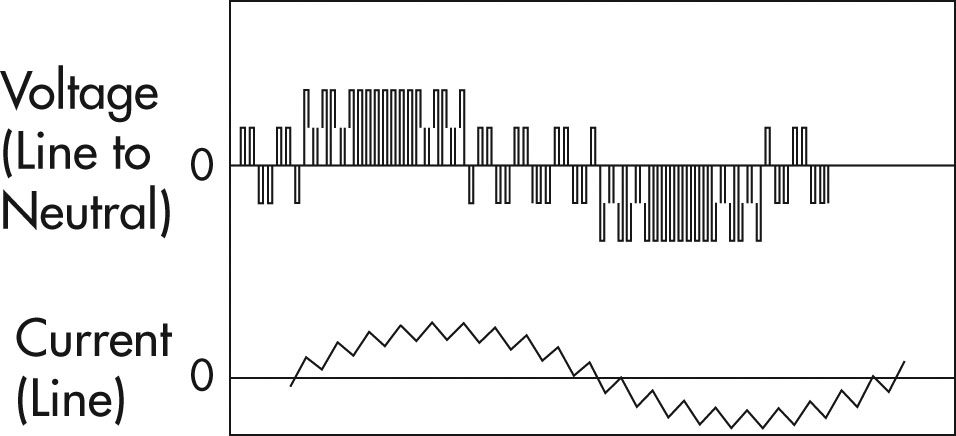# Principles of Operation - AC VFD Drives

## a. AC Induction Motors

An AC induction motor is constructed with a rotor that has windings which intersect the rotating magnetic field generated by the stator windings.

At full load speed, the rotor turns slightly slower than the synchronous speed of the motor. This is because the magnetic field causes currents to flow in the rotor windings and produces a torque which turns the rotor; so if the rotor turns at the same speed as the magnetic field, there would be no relative motion between the rotor and the magnetic field, and no torque would be produced.

The amount of speed by which the rotor lags the rotating magnetic field is known as the slip of the motor. The higher the slip, the more torque is produced by the motor.

The speed at which the magnetic field rotates depends on the number of poles or coils distributed around the stator and the frequency of the supply current. This is called the synchronous speed.

Synchronous Speed = 120 x Frequency
Number of poles

Typical AC induction motor speeds are 3600, 1800, 1200, and 900 RPM.

The following diagram shows the torque-speed relationship of a typical induction motor.

Text version: Figure 4

## Figure 4

Graph with starting torque on the vertical axis going from 0 to 200 and % speed on the horizontal axis going from 0 to 100.

Plotted line starts at 160 starting torque and 0% speed and curves downward to 125 starting torque at 25% speed where it begins to curve back upwards until it reaches at peak of 200 starting torque at 75% speed. The plotted line then drops down to 0 starting torque at 100% speed. The initial downward curve is labelled as "Pull-up Torque" and the drop off after the peak is labelled as "Breakdown Torque".

## b. Squirrel Cage AC Induction Motors

Most AC induction motors are squirrel cage motors.

The rotor windings in a squirrel cage motor are aluminum or copper alloy bars that are positioned along the direction of the shaft and short circuited by end rings as shown in the following diagram.

The shape of the bars and the resistance of the alloy used in their construction influences the torque-speed characteristics of the motor.

## c. Pulse Width Modulated Variable Frequency Drives

When operated from a constant frequency power source (typically 60Hz), AC induction motors are fixed speed devices.

A variable frequency drive controls the speed of an AC motor by varying the frequency supplied to the motor.

The drive also regulates the output voltage in proportion to the output frequency to provide a relatively constant ratio of voltage to frequency (V/Hz), as required by the characteristics of the AC motor to produce adequate torque.

The first step in this process is to convert the AC supply voltage into DC by the use of a rectifier. DC power contains voltage ripples which are smoothed using filter capacitors. This section of the VFD is often referred to as the DC link.

This DC voltage is then converted back into AC. This conversion is typically achieved through the use of power electronic devices such as IGBT power transistors using a technique called Pulse Width Modulation (PWM). The output voltage is turned on and off at a high frequency, with the duration of on-time, or width of the pulse, controlled to approximate a sinusoidal waveform.

Older drive technologies like Current Source Inverters and Variable Voltage Controllers used SCRs or Thyristors as control devices. These technologies have now been replaced by the PWM VFD.

The entire process is controlled by a microprocessor which monitors the:

• incoming voltage supply,
• speed set-point,
• output voltage and current to ensure operation of the motor within established parameters.Graph comparing voltage to current for a Pulse Width Modulated Variable Frequency Drive